# 3 Finite element implementation

$$3.1 IntroductionThe CSFM considers continuous stress fields in the concrete (2D finite elements), complemented by discrete “rod” elements representing the reinforcement (1D finite elements). Therefore, the reinforcement is not diffusely embedded into the concrete 2D finite elements, but explicitly modeled and connected to them. A plane stress state is considered in the calculation model.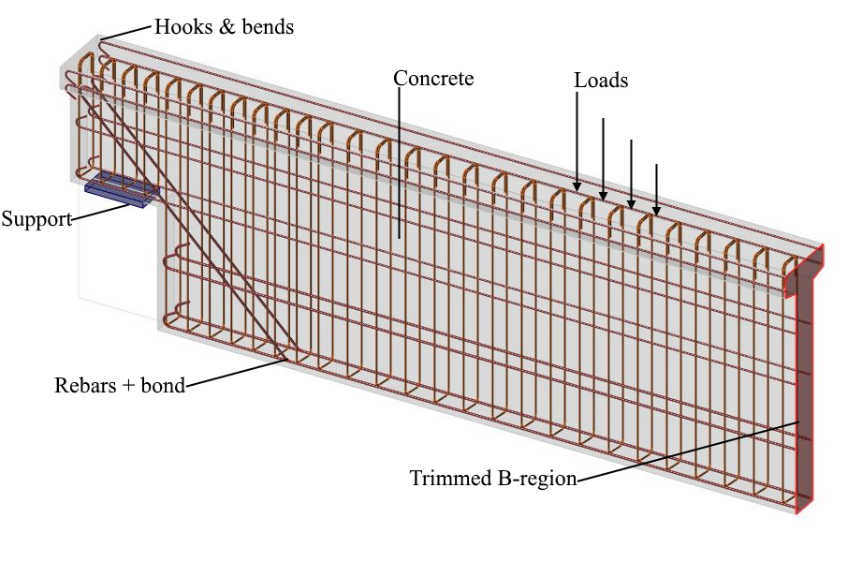$\textsf{\textit{\footnotesize{Fig. 8\qquad Visualization of the calculation model of a structural element (trimmed beam) in Idea StatiCa Detail.}}}$Both entire walls and beams, as well as details (parts) of beams (isolated discontinuity region, also called trimmed end), can be modeled. In the case of walls and entire beams, supports must be defined in such a way that an (externally) isostatic (statically determinate) or hyperstatic (statically indeterminate) structure results. The load transfer at the trimmed ends of beams is introduced by means of a special Saint-Venant transfer zone (described in Section 3.3), which ensures a realistic stress distribution in the analyzed detail region.3.2 Supports and load transmitting componentsTo model most of the situations during the construction process, many types of supports (Fig. 9) and components used for transferring load (Fig. 10) are available in the CSFM.3.2.1 SupportsPoint support can be modeled in several ways to ensure that stresses are not localized in one point but rather distributed over a larger area. The first option is a distributed point support (Fig. 9a), which uniformly distributes the load on the edge of member over the specified width.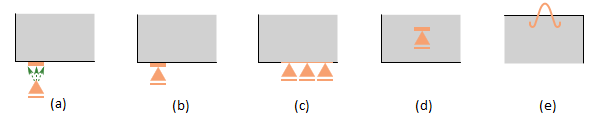$\textsf{\textit{\footnotesize{Fig. 9\qquad Various types of supports:}}}$$\textsf{\textit{\footnotesize{(a) point distributed; (b) bearing plate; (c) line support; (d) patch support; (e) hanging.}}}$Patch support (Fig. 9d), on the other hand, can only be placed inside a volume of concrete with a defined effective radius. It is then connected by rigid elements to the nodes of the reinforcement mesh within this radius. Therefore, it is required to define a reinforcing cage around patch support.For the more precise modeling of some real scenarios, there are two other options for point supports. Firstly, there is point support with a bearing plate of defined width and thickness (Fig. 9b). The material of the bearing plate can be specified, and the whole bearing plate is meshed independently. Secondly, there is hanging support available (Fig. 9e), which can be used for modeling lifting anchors or lifting studs.Line support (Fig. 9c) can be defined on an edge (by specifying its length) or inside an element (by a polyline). It is also possible to specify its stiffness and/or non-linear behavior (support in compression/tension or only in compression).3.2.2 Load transmitting componentsThe introduction of loads into the structure can also be modeled in several ways. For point loads, a bearing plate (Fig. 10a) can be used similarly as point support, distributing the concentrated load onto a larger area thanks to steel plate with defined width and thickness. Patch loads (Fig. 10b and Fig. 11) are placed inside the concrete with a certain effective radius and are connected by rigid elements to the nodes of nearby reinforcing bars.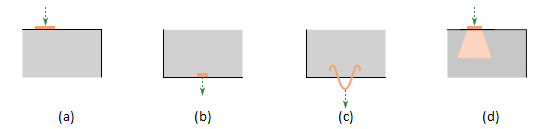$\textsf{\textit{\footnotesize{Fig. 10\qquad Various types of load transfer components:}}}$$\textsf{\textit{\footnotesize{(a) bearing plate; (b) patch load; (c) hanging; (d) partially loaded area.}}}$Lifting anchors or lifting studs can be modeled by a hanging load (Fig. 10c). User can use partially loaded area (Fig. 10d), which allows increasing load-bearing capacity of concrete in compression according to Eurocode (it is not possible to use this type of load transmitting component when ACI is set). The structure can also be loaded with line loads on the edges, by general polyline or by surface loads, representing, e.g., self-weight (which is not automatically considered in the analysis).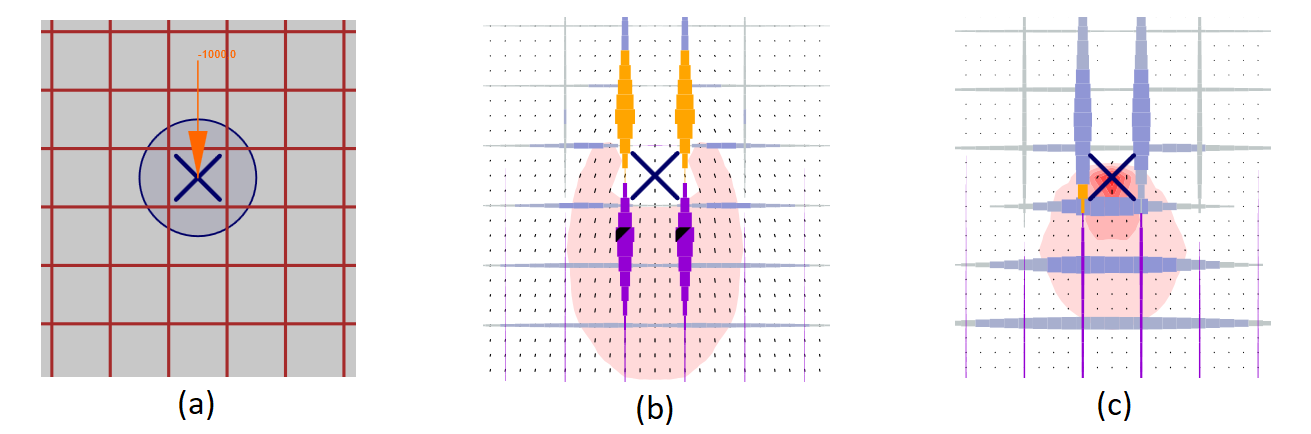$\textsf{\textit{\footnotesize{Fig. 11\qquad Patch load: (a) load application; (b) load transferred through rebars; (c) load transferred through concrete.}}}$3.3 Load transfer at trimmed ends of beamsIn many cases, we need to model only some detail (part) of a structural member such as beam support, opening in the middle of the beam, etc. This approach can lead to support configurations that are unstable but admissible in Idea StatiCa Detail (including the case of no supports). However, in such cases, it is also necessary to model the section representing the connection to the adjoining B-region, including internal forces at this section which satisfy the equilibrium. In certain cases (e.g., when modeling beam support), these internal forces can be determined automatically by the program.Between B-region and the analyzed discontinuity region, a Saint-Venant transfer zone is automatically created to ensure a realistic stress distribution in the analyzed region. The width of the transfer zone is determined as half of the section’s depth. As the only purpose of the Saint-Venant zone is to achieve a proper stress distribution in the rest of the model, no results from this area are displayed in verification, and no stop criteria are considered here.The edge of the Saint-Venant zone that represents the trimmed end of the beam is modeled as rigid, i.e., it may rotate, but must rest plane. This is done by connecting all the FEM nodes of the edge to a separate node at the center of inertia of the section using a rigid body element (RBE2). The internal forces of the element may then be applied at this node, as shown in Fig. 12.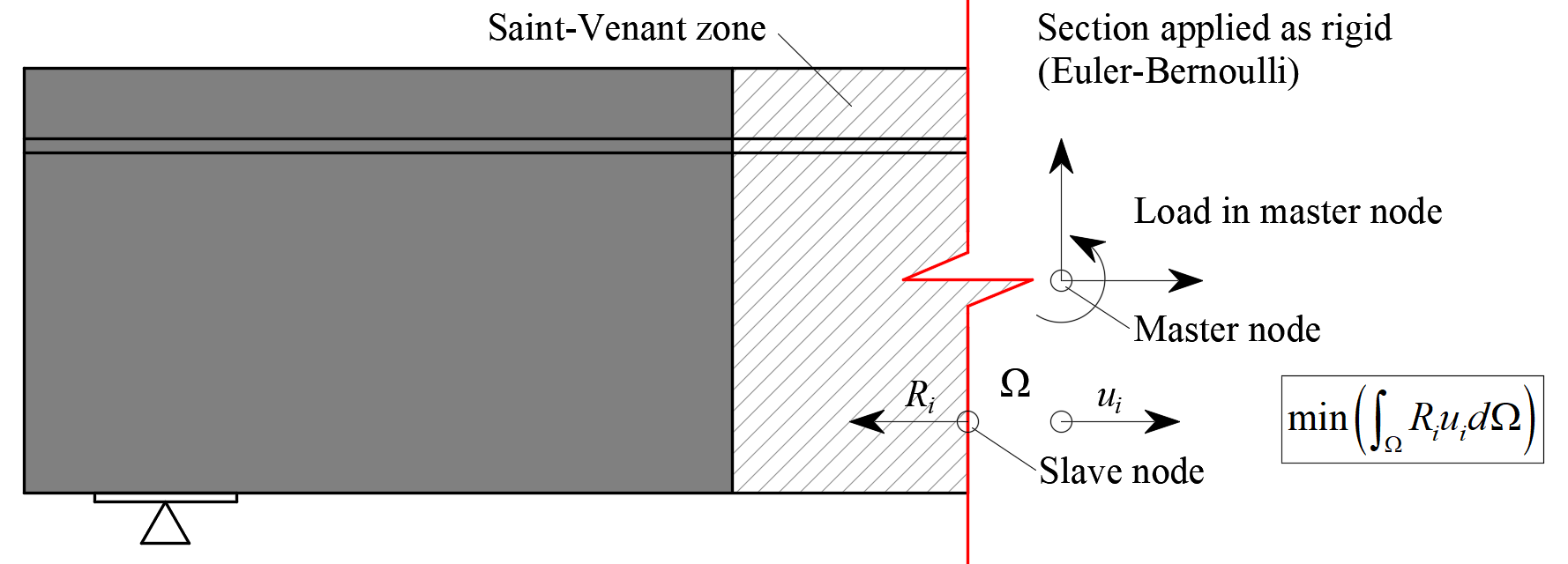$\textsf{\textit{\footnotesize{Fig. 12\qquad Transfer of internal forces at a trimmed end.}}}$3.4 Geometric modification of cross-sectionsIn the case of geometries which include haunches, the width of the wall elements used to model the haunch is reduced in comparison to the original width so that it is equal to double its height plus the thickness of the adjacent wall. This is based on the assumption that a compression stress field would expand from the wall at a 45° angle (see Fig. 13), so the aforementioned reduced width would be the maximal width capable of transferring loads.Note that the method of determining effective width flange implemented in CSFM is different from the one stated in 5.3.2.1 EN 1992-1-1 (2015). Besides geometry, Eurocode based effective width flange is explicitly affected by the span lengths and boundary conditions of a structure.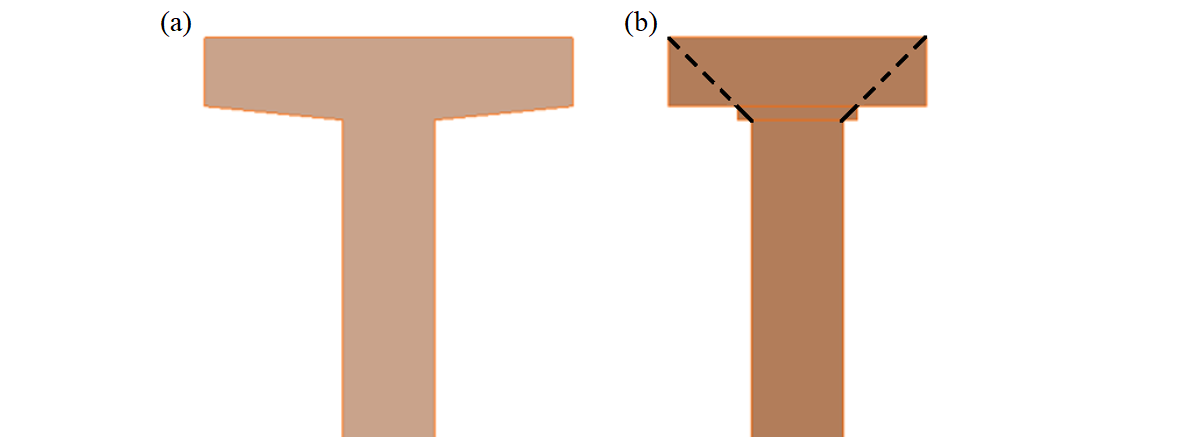$\textsf{\textit{\footnotesize{Fig. 13\qquad Width reduction of a cross-section: (a) user input; (b) FE model – automatically determined reduced flange width.}}}$In the case of haunches lying in the horizontal plane (Fig. 14), each haunch is divided into five sections along its length. Each of these sections is then modeled as a wall with a constant thickness, which is equal to the real thickness in the middle of the respective section.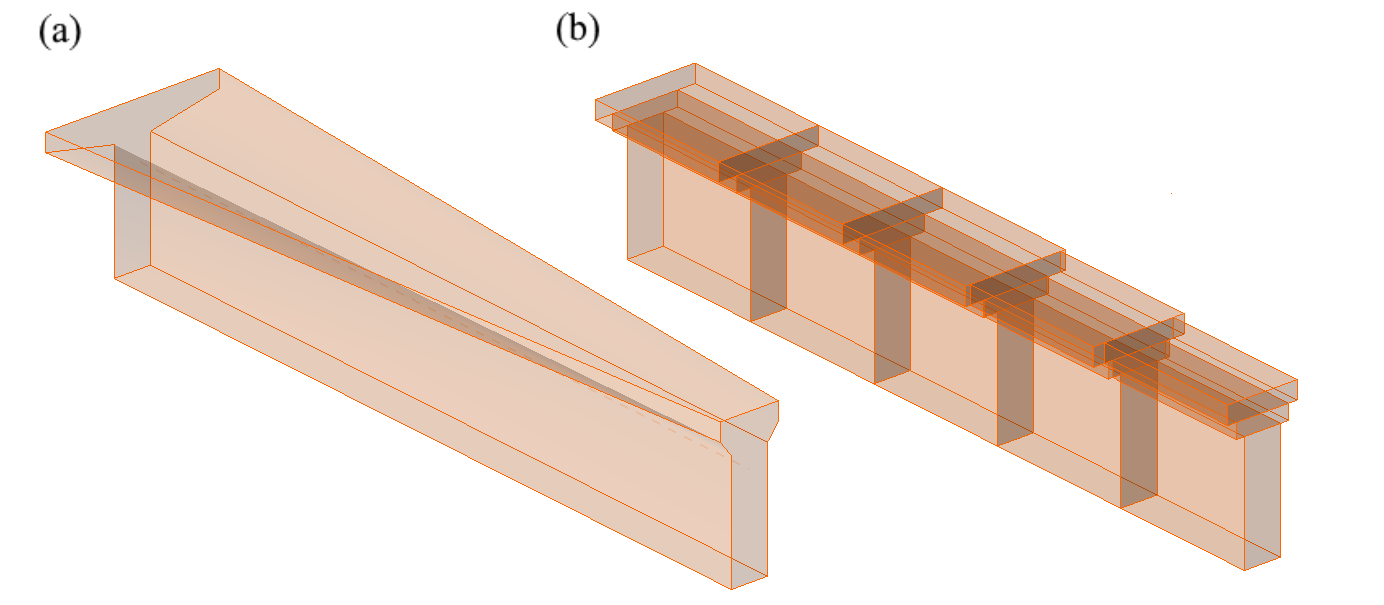$\textsf{\textit{\footnotesize{Fig. 14\qquad Horizontal haunch: (a) user input; (b) FE model – a haunch automatically divided into five sections.}}}$3.5 Finite element typesThe non-linear finite element analysis model is created by several types of finite elements used to model concrete, reinforcement, and the bond between them. Concrete and reinforcement elements are first meshed independently and then connected to each other using multi-point constraints (MPC elements). This allows the reinforcement to occupy an arbitrary, relative position in relation to the concrete. If anchorage length verification is to be calculated, bond and anchorage end spring elements are inserted between the reinforcement and the MPC elements.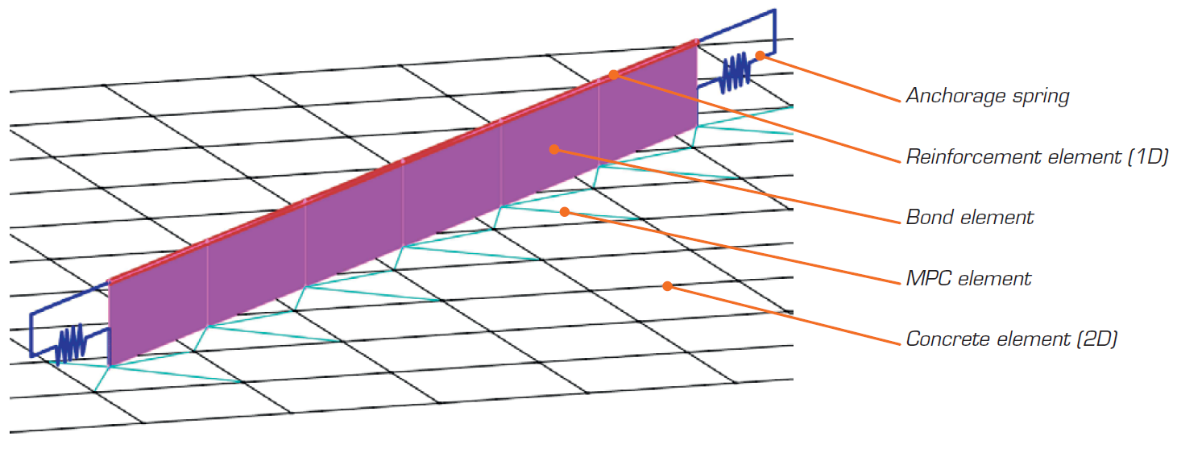$\textsf{\textit{\footnotesize{Fig. 15\qquad Finite element model: reinforcement elements mapped to concrete mesh using MPC elements and bond elements.}}}$3.5.1 ConcreteConcrete is modeled using quadrilateral and trilateral shell elements, CQUAD4 and CTRIA3. These can be defined by four or three nodes, respectively. Only plane stress is assumed to exist in these elements, i.e., stresses or strains in the z-direction are not considered.Each element has four or three integration points which are placed at approximately 1/4 of its size. At each integration point in every element, the directions of principal strains α1, α3 are calculated. In both of these directions, the principal stresses σc1, σc3 and stiffnesses E1, E2 are evaluated according to the specified concrete stress-strain diagram, as per Fig. 2. It should be noted that the impact of the compression softening effect couples the behavior of the main compressive direction to the actual state of the other principal direction.3.5.2 ReinforcementRebars are modeled by two-node 1D “rod” elements (CROD), which only have axial stiffness. These elements are connected to special “bond” elements which were developed in order to model the slip behavior between a reinforcing bar and the surrounding concrete. These bond elements are subsequently connected by MPC (multi-point constraint) elements to the mesh representing the concrete. This approach allows the independent meshing of reinforcement and concrete, while their interconnection is ensured later.3.5.3 Anchorage length verification: bond elementsThe anchorage length is verified by implementing the bond shear stresses between concrete elements (2D) and reinforcing bar elements (1D) in the finite element model. To this end, a “bond” finite element type was developed.The definition of the bond element is similar to that of a shell element (CQUAD4). It is also defined by 4 nodes, but in contrast to a shell, it only has a non-zero stiffness in shear between the two upper and two lower nodes. In the model, the upper nodes are connected to the elements representing reinforcement and the lower nodes to those representing concrete. The behavior of this element is described by the bond stress, τb, as a bilinear function of the slip between the upper and lower nodes, δu, see Fig. 16.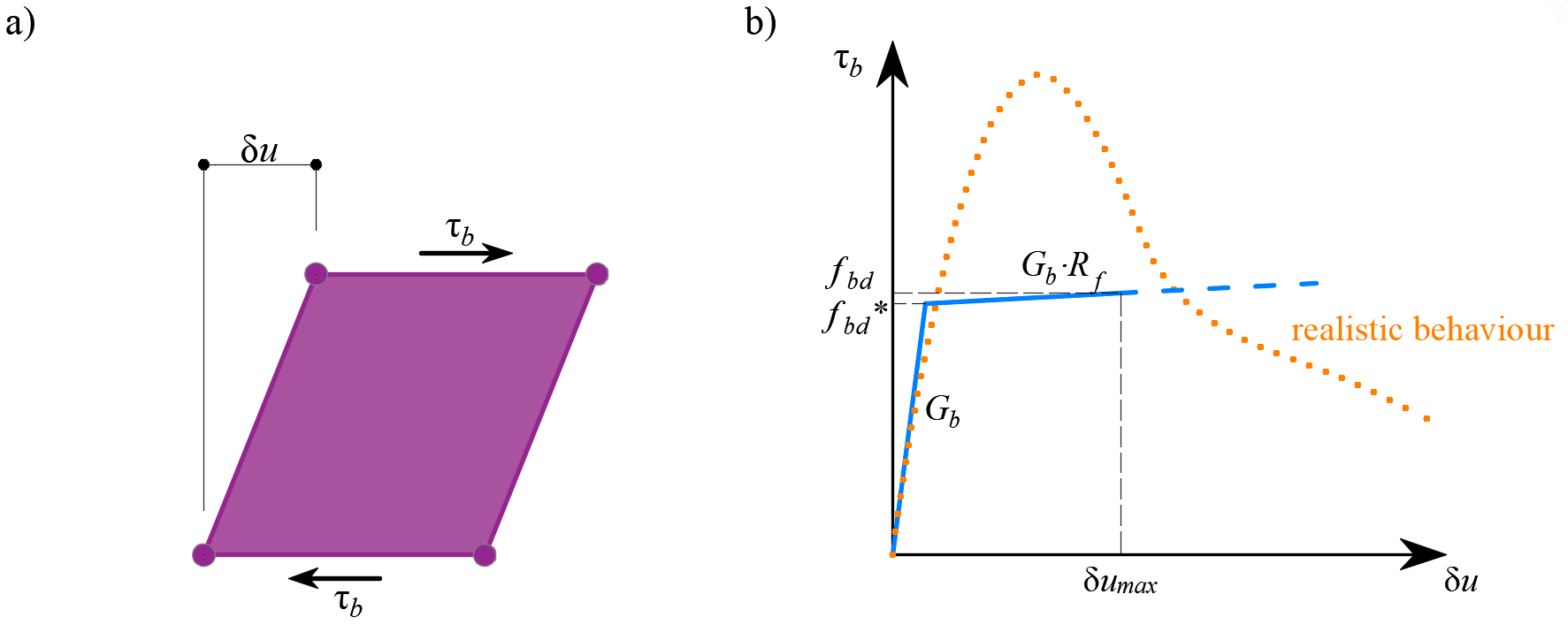$\textsf{\textit{\footnotesize{Fig. 16\qquad (a) conceptual illustration of the deformation of a bond element; (b) a stress-deformation function.}}}$The elastic stiffness modulus of the bond-slip relationship, Gb, is defined as follows:$G_b = k_g \cdot \frac{E_c}{Ø}$where:kg coefficient depending on the reinforcing bar surface (by default kg = 0.2),Ec modulus of elasticity of concrete, taken as EcmØ the diameter of the reinforcing bar.The design values of ultimate bond shear stress, fbd, provided in the respective selected design codes EN 1992-1-1 (2015) or ACI 318-04 are used to verify the anchorage length. The hardening of the plastic branch is calculated by default as Gb/105.3.5.4 Anchorage length verification: spring elementsThe provision of anchorage ends to the reinforcing bars (i.e., bends, hooks, loops…), which fulfills the prescriptions of design codes, allows the reduction of the basic anchorage length of the bars (lb,net) by a certain factor β (referred to as the ‘anchorage coefficient’ below). The design value of the anchorage length (lb) is then calculated as follows:$l_b = \left(1 - \beta\right)l_{b,net}$The available anchorage types in the CSFM include a straight bar (i.e., no anchor end reduction), bend, hook, loop, welded transverse bar, perfect bond and continuous bar. All these types, along with the respective anchorage coefficients β, are shown in Fig. 17 for longitudinal reinforcement and in Fig. 18 for stirrups. The values of the adopted anchorage coefficients are in accordance with EN 1992-1-1. It should be noted that in spite of the different available options, the CSFM just distinguishes three types of anchorage ends: (i) no reduction in the anchorage length, (ii) a reduction of 30 % of the anchorage length in the case of a normalized anchorage and (iii) perfect bond.$\textsf{\textit{\footnotesize{Fig. 17\qquad Available anchorage types and respective anchorage coefficients for longitudinal reinforcing bars in the CSFM:}}}$$\textsf{\textit{\footnotesize{(a) straight bar; (b) bend; (c) hook; (d) loop; (e) welded transverse bar; (f) perfect bond; (g) continuous bar.}}}$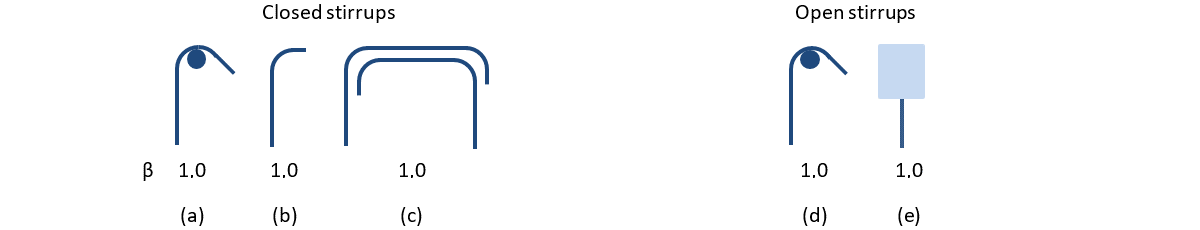$\textsf{\textit{\footnotesize{Fig. 18\qquad Available anchorage types and respective anchorage coefficients for stirrups.}}}$$\textsf{\textit{\footnotesize{Closed stirrups: (a) hook; (b) bend; (c) overlap. Open stirrups: (d) hook; (e) continuous bar.}}}$The intended reduction in lb,net is equivalent to the activation of the reinforcing bar at its end at a percentage of its maximum capacity given by the anchorage reduction coefficient, as shown in Fig. 19a.$\textsf{\textit{\footnotesize{Fig. 19\qquad Model for the reduction of the anchorage length:}}}$$\textsf{\textit{\footnotesize{(a) anchorage force along the anchorage length of the reinforcing bar; (b) slip-anchorage force constitutive relationship.}}}$The reduction of the anchorage length is included in the finite element model by means of a spring element at the end of the bar (Fig. 15), which is defined by the constitutive model shown in Fig. 19b. The maximum force transmitted by this spring (Fau) is:$F_{au} = \beta \cdot A_s \cdot f_{yd}$where :β the anchorage coefficient based on anchorage type,As the cross-section of the reinforcing bar,fyd the design value of the yield strength of the reinforcement.3.6 MeshingThe finite elements described in the previous chapter are implemented internally, and the analysis model is generated automatically without any need for proficient user interaction. An important part of this process is meshing.3.6.1 ConcreteAll concrete members are meshed together. A recommended element size is automatically computed by the application based on the size and shape of the structure and taking into account the diameter of the largest reinforcing bar. Moreover, the recommended element size guarantees that a minimum of 4 elements are generated in thin parts of the structure, such as slim columns or thin slabs, to ensure reliable results in these areas. The maximum number of concrete elements is limited to 5000, but this value is sufficient to provide the recommended element size for most structures. Designers can always select a user-defined concrete element size by modifying the multiplier of the default mesh size.3.6.2 ReinforcementThe reinforcement is divided into elements with approximately the same length as the concrete element size. Once the reinforcement and concrete meshes are generated, they are interconnected with bond elements (ULS) or directly by MPC elements (SLS), as shown in Fig. 15.3.6.3 Bearing platesAuxiliary structural parts, such as bearing plates, are meshed independently. The size of these elements is calculated as 2/3 of the size of concrete elements in the connection area. The nodes of the bearing plate mesh are then connected to the edge nodes of the concrete mesh using interpolation constraint elements (RBE3).3.6.4 Loads and supportsPatch loads and patch supports are connected only to the reinforcement, as shown in Fig. 20. Therefore, it is necessary to define the reinforcement around them. Connection to all nodes of the reinforcement within the effective radius is ensured by RBE3 elements with equal weight.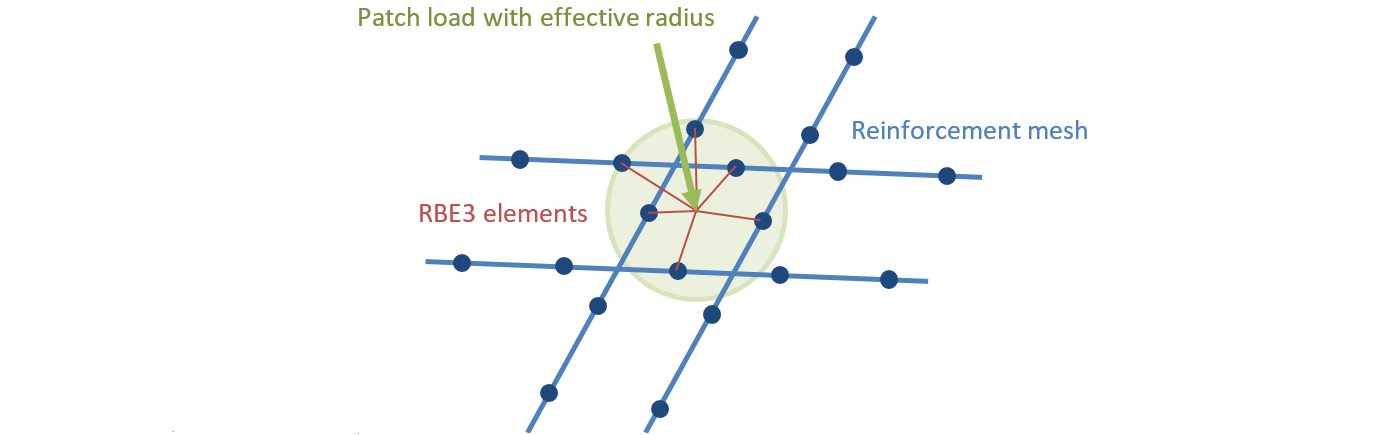$\textsf{\textit{\footnotesize{Fig. 20\qquad Patch load mapping to reinforcement mesh.}}}$Line supports and line loads are connected to the nodes of the concrete mesh using RBE3 elements based on the specified width or effective radius. The weight of the connections is inversely proportional to the distance from the support or load impulse.3.7 Solution method and load-control algorithmA standard full Newton-Raphson (NR) algorithm is used to find the solution to a non-linear FEM problem. The implementation is almost identical to the one presented in .Generally, the NR algorithm does not often converge when the full load is applied in a single step. A usual approach, which is also used here, is to apply the load sequentially in multiple increments and use the result from the previous load increment to start the Newton solution of a subsequent one. For this purpose, a load control algorithm was implemented on top of the Newton-Raphson. In the case that the NR iterations do not converge, the current load increment is reduced to half its value and the NR iterations are retried.A second purpose of the load-control algorithm is to find the critical load, which corresponds to certain “stop criteria” – specifically the maximum strain in concrete, the maximum slip in bond elements, the maximum displacement in anchorage elements and the maximum strain in reinforcing bars. The critical load is found using the bisection method. In the case that the stop criterion is exceeded anywhere in the model, the results of the last load increment are discarded, and a new increment of half the size of the previous one is calculated. This process is repeated until the critical load is found with a certain error tolerance.For concrete, the stop criterion was set by default to a 5% strain in compression (i.e., around an order of magnitude larger than the actual failure strain of concrete) and 7% in tension at the integration points of shell elements. In tension, the value was set to allow for the limit strain in reinforcement, which is usually around 5% without accounting for tension stiffening, to be reached first. In compression, the value was chosen from among several alternatives, as one that is large enough for the effects of crushing to be visible in the results, but small enough so as not to cause too many problems with numerical stability.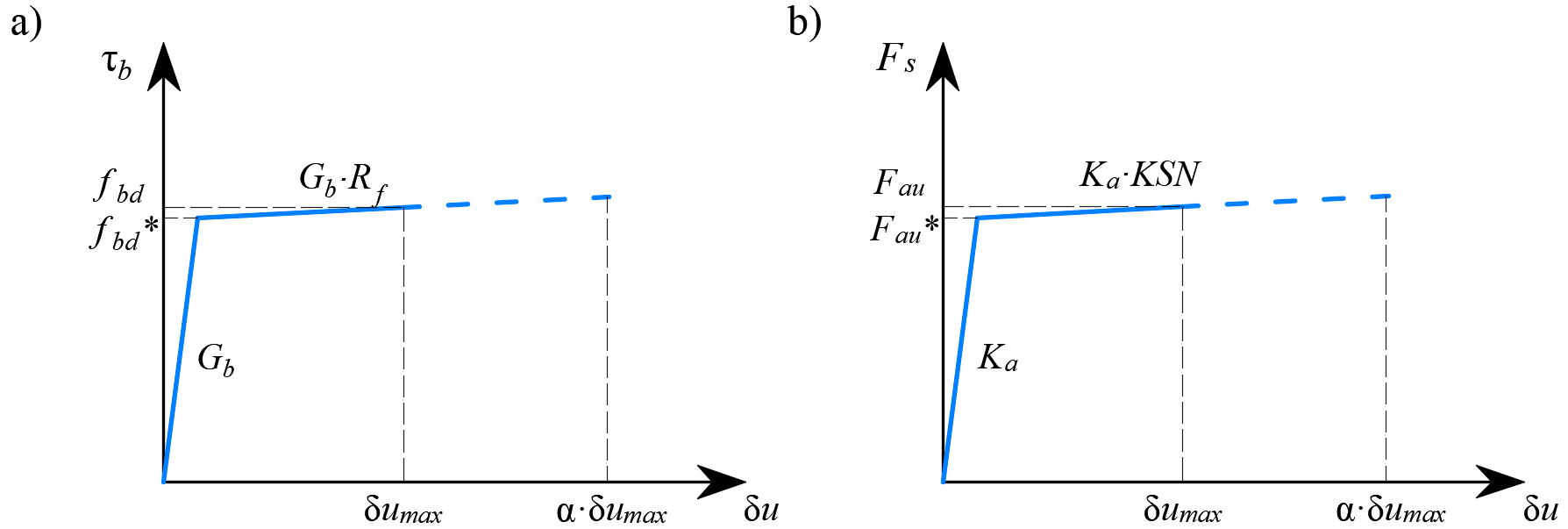$\textsf{\textit{\footnotesize{Fig. 21\qquad Constitutive relationship of bond and anchorage elements used for anchorage length verification:}}}$$\textsf{\textit{\footnotesize{(a) bond shear stress slip response of a bond element; (b) force-displacement response of an anchorage element.}}}$For reinforcement, the stop criterion is defined in terms of stresses. Since stresses at the crack are modeled, the criterion in tension corresponds to the reinforcement tensile strength accounting for the safety coefficient. The same value is used for the criterion in compression.The stop criterion in bond and anchorage elements is α·δumax, where δumax is the maximal slip used in code checks and α = 10.3.8 Presentation of resultsResults are presented independently for concrete and for reinforcement elements. The stress and strain values in concrete are calculated at the integration points of shell elements. However, as it is not practical to present the data in such a manner, the results are presented by default in nodes, like the maximal value of compressive stress from adjacent gauss integration points in connected elements (Fig. 22). It should be noted that this representation might locally underestimate the results at compressed edges of members in a case that the finite-element size is similar to the depth of the compression zone.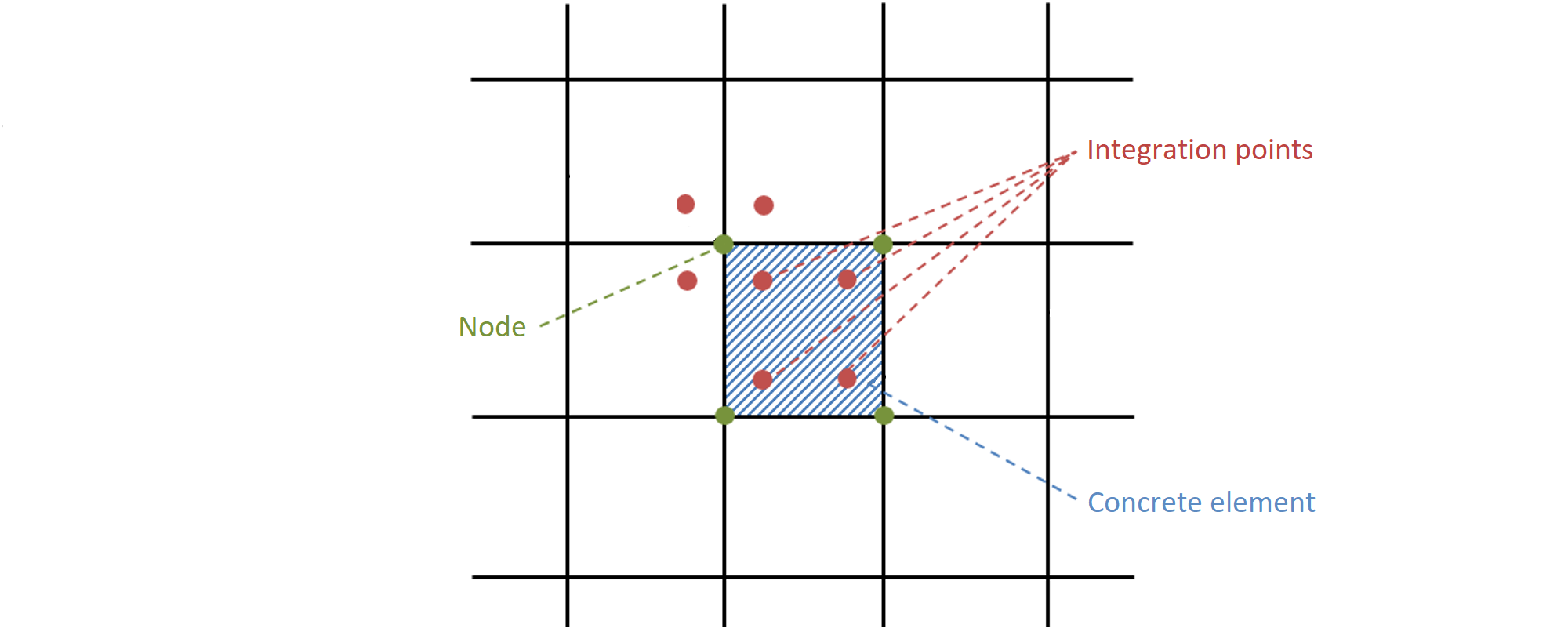$\textsf{\textit{\footnotesize{Fig. 22\qquad Concrete finite element with integration points and nodes: presentation of the results for concrete in nodes and}}}$$\textsf{\textit{\footnotesize{in finite elements.}}}$The results for the reinforcement finite elements are either constant for each element (one value – e.g., for steel stresses) or linear (two values – for bond results). For auxiliary elements, such as elements of bearing plates, only deformations are presented.$$